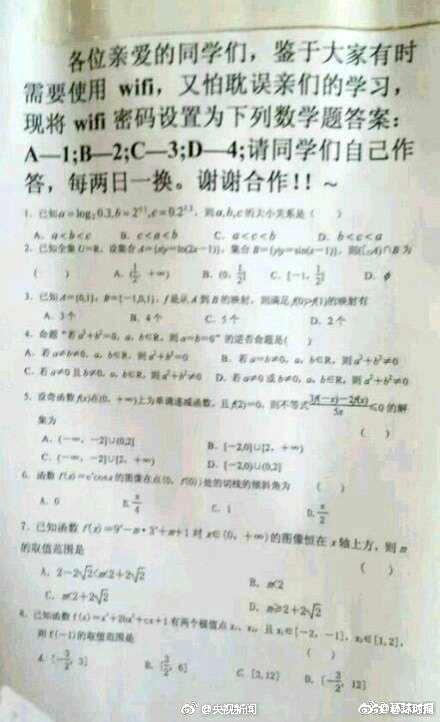### 题目描述：8
A-T B-F C-F D-F
C-T B-F A-F D-F
A-F D-F C-F B-T
B-T A-F C-F D-F
B-F D-T A-F C-F
A-T C-F B-F D-F
D-T B-F C-F A-F
C-T A-F B-F D-F

13224143

### AC代码：

``````for i in range(eval(input())):
s = input()
print(ord(s[s.find('T')-2])-ord('A')+1,end="")``````